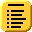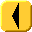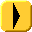## Introduction to Programming and C++The auto increment operator ++ may be used before or after the variable being incremented. If the auto increment is the only part of a statement, then there is no difference. This is the preferable usage of ++, as demonstrated in Program 1.

Program 1. i++ and ++i as individual statements.

 ```#include using namespace std; int main() { int i = 10; i++; ++i; cout << "The value of 'i' is " << i << endl; return 0; } ```

If, however, you use the result of the auto increment, e.g., you assign the result to another variable, you get different results depending on which you use:

++i
This increments the variable i (the ++ comes first) and then returns the result.
i++
This stores the current value of i (before the increment), then increments the value variable i, but returns the original value.

Program 2 demonstrates the use of ++i, while Program 3 demonstrates i++.

Program 2. Demonstration of ++i.

 ```#include using namespace std; int main() { int i = 10; int n = ++i; // n is assigned 11 (the new value of i) cout << "The value of 'i' is " << i << endl; cout << "The value of 'n' is " << n << endl; return 0; } ```

Program 3. Demonstration of i++.

 ```#include using namespace std; int main() { int i = 10; int n = i++; // n is assigned 10 (the original value of i) cout << "The value of 'i' is " << i << endl; cout << "The value of 'n' is " << n << endl; return 0; } ```

These harken back to the days where programmers' abilities to generate optimized code far exceeded the abilities of the compiler. Programs 4 and 5 demonstrate one example where an appropriate use of ++ may be useful. In Program 5, the pointer is simply incremented with each step, while in Program 4, the location of the array must be calculated with each loop.

Program 4. Looping through an array.

 ```#include using namespace std; int main() { const int N = 10000; int array[N]; for ( int j = 0; j < N; ++j ) { for ( int i = 0; i < N; ++i ) { array[i] = i+i; } } cout << "array = " << array << endl; return 0; } ```

Program 5. Faster looping through an array.

 ```#include using namespace std; int main() { const int N = 10000; int array[N]; for ( int j = 0; j < N; ++j ) { for ( int i = 0, * ptr = array; i < N; ++i ) { *(ptr++) = i+j; } } cout << "array = " << array << endl; return 0; } ```

In general, however, do not use the return value of ++. It may seem clever and cute, but if you ask more inexperienced C++ users to explain the difference or even worse, correct a bug resulting from an incorrect usage of the return value of ++, you will quickly find there are significant draw-backs. Besides, most optimizers will fix any such weaknesses.

# One of Java's Sore Spots...

When I was teaching ECE 250 using Java, I was very embarrassed to find that the default Java compiler could not determine that i++; was a single statement on a single line and still kept the return value even if it was not going to be used. The g++, fortunately, is a little more up to date.

# Questions

1. Compile Programs 2 and 3 and compare the size of the resulting executables. Is there a difference?

2. Take Program 5 and explain what happens if you replace the line

```	*(ptr++) = i+j;
```

with

```	*(++ptr) = i+j;
```

There are two bugs: one logical, the other possibly being the source of a crash.Top